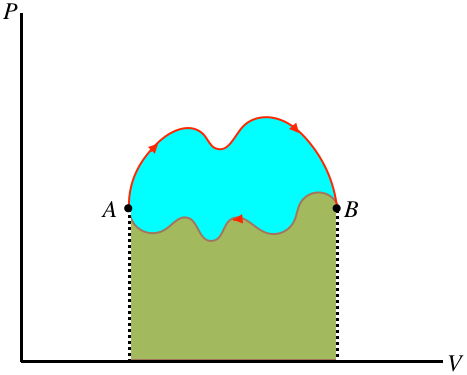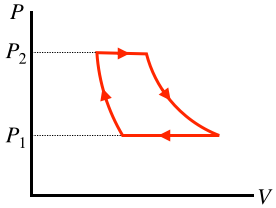$$\require{cancel}$$

# 6.1: Cyclic Processes

One process that we will discuss in detail later is called a cyclic process. Simply put, this is a process that returns to the same thermodynamic state at which it started. In a process diagram, it forms a closed loop:

Figure 6.1.1 – A Cyclic ProcessOne of the state variables that returns to its original value when the cycle is complete is the internal energy. This means that for a full cycle we can use the first law to conclude:

$0=\Delta U = Q - W \;\;\;\Rightarrow\;\;\; Q=W$

If there is a net amount of heat that comes into the system, it goes out of the system in the form of work – heat enters the gas, which in turn expands and does work on the piston. On the other hand, if a net amount of heat exits the system, it must have entered in the form of work. We can in fact even draw a general conclusion about which of these is occurring (and how much energy has been converted), based on the direction of the cycle (clockwise or counterclockwise) in the $$PV$$ diagram. Whichever direction the cycle goes, part of the process is going left-to-right, and the rest is going right-to-left. The section of the process that is higher has a greater area under the curve, so if the top section is left-to-right there is net work done by the gas, and if it is right-to-left there is net work on the gas. Furthermore, the difference in the right-going and left-going processes is the net work done, and this is the area bounded by the cyclic loop.

Figure 6.1.2 – Work Done in a Cyclic Process is the Area Bounded by the CycleIn the figure above, the area under the upper portion of the curve is positive, and the area under the lower portion is negative, so the total area for the full process is the difference, which is the blue region enclosed by the loop defining the cycle. Notice that for the case shown, the total area is positive, and if the cycle went the other way then the net work would be negative. We therefore conclude:

• the area inside a closed cycle loop in a $$PV$$ diagram represents the net amount of energy converted between work and heat
• clockwise cyclic processes on the $$PV$$ diagram represent physical systems for which heat is entering and an equal amount of work is done by the gas
• counterclockwise cyclic processes on the $$PV$$ diagram represent physical systems for which work is done on the gas and an equal amount of heat is expelled by the gas

Example $$\PageIndex{1}$$

The cyclic process of an ideal gas shown in the $$PT$$ diagram below is rectangular, with vertical and horizontal sides at the positions labeled.1. Find the total heat transferred during the full cycle in terms of the quantities labeled, and indicate whether the heat is transferred into or out of the system.
2. Sketch the $$PV$$ diagram of this cyclic process.
Solution

a. As this is not a $$PV$$ diagram, the total work done or heat exchanged is not simply the area inside the closed shape. We therefore need to look at each segment individually. The horizontal segments are processes that maintain a constant pressure, so they are isobaric. We know the work done during such a process in terms of the temperature change from Equation 5.8.9: $$Q=nC_P\Delta T$$. The two isobaric processes involve equal/opposite temperature changes, so these heat exchanges cancel each other out. The vertical segments are processes that maintain a constant temperature, so they are isothermal, and we can write down the work done for each segment using Equation 5.8.16:

$\left. \begin{array}{l} left\; segment: && Q = nRT_1\ln\left[\dfrac{P_1}{P_2}\right] \\ right\; segment: && Q = nRT_2\ln\left[\dfrac{P_2}{P_1}\right] \end{array} \right\} \;\;\; \Rightarrow \;\;\; Q_{tot} = nRT_2\ln\left[\dfrac{P_2}{P_1}\right] + nRT_1\ln\left[\dfrac{P_1}{P_2}\right] = nR\left(T_2-T_1\right)\ln\left[\dfrac{P_2}{P_1}\right] \nonumber$

b. We need to sketch two isobaric and two isothermal processes that form a closed loop in a $$PV$$ diagram. The isotherms need to be hyperbolas, which means the upper corners need to be at lower volumes than the corners directly below them on the $$PT$$ diagram. Also, the work done in the upper isobaric process has to be equal in magnitude to the work done in the lower isobaric process (since we found that they cancel in part a). But the upper process is at a higher pressure, so the volume change has to be smaller (i.e. the horizontal segment is shorter on top than on bottom).